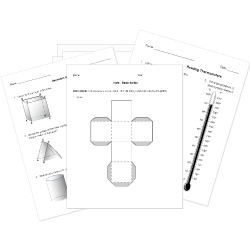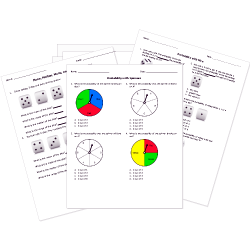Tweet# Printable Elementary Math Worksheets, Tests, and Activities

Assess student understanding of arithmetic and geometry concepts with these math worksheets for K-5 students.

Worksheets labeled with are accessible to Help Teaching Pro subscribers only. Become a Pro Subscriber to access hundreds of standards aligned worksheets.

## Daily Math Practice Printables

Supplement your lower elementary curriculum and reinforce essential math skills with our daily math practice worksheets. Each week features a two-page worksheet designed for front-to-back printing while providing space for student work. Use the four days of math problems for morning work or weeknight homework. Separate fifth day quizzes are included for optional progress monitoring. Worksheets are Common Core aligned and offer spiral review of previously covered standards. Select the worksheet link to view the alignment note in the upper left corner of its page. Review weeks may be used anytime during the school year to meet your teaching needs or as summer math practice.

### General Practice Printables

Use these worksheets with any of the daily math practice printables found below.

### Constructive Response Practice and Review Worksheets

These tests feature questions modeled after PARCC Mathematics items. Search for PARCC questions to see all content available.

## Elementary ArithmeticPractice arithmetic skills with this collection of free math number sense worksheets for addition, subtraction, multiplication, division, fractions, and decimals. Answer pictograph questions for basic addition, subtraction, and fraction problems.

## Elementary Geometry and MeasurementInvestigate lines, angles, circles, triangles, polygons, perimeter, volume, and the coordinate plane, among other topics with these geometry worksheets. Print net activities (3D models) for hands-on student learning.

### KindergartenElementary students can begin to develop statistical thinking by learning to read and interpret data presented in graphs.